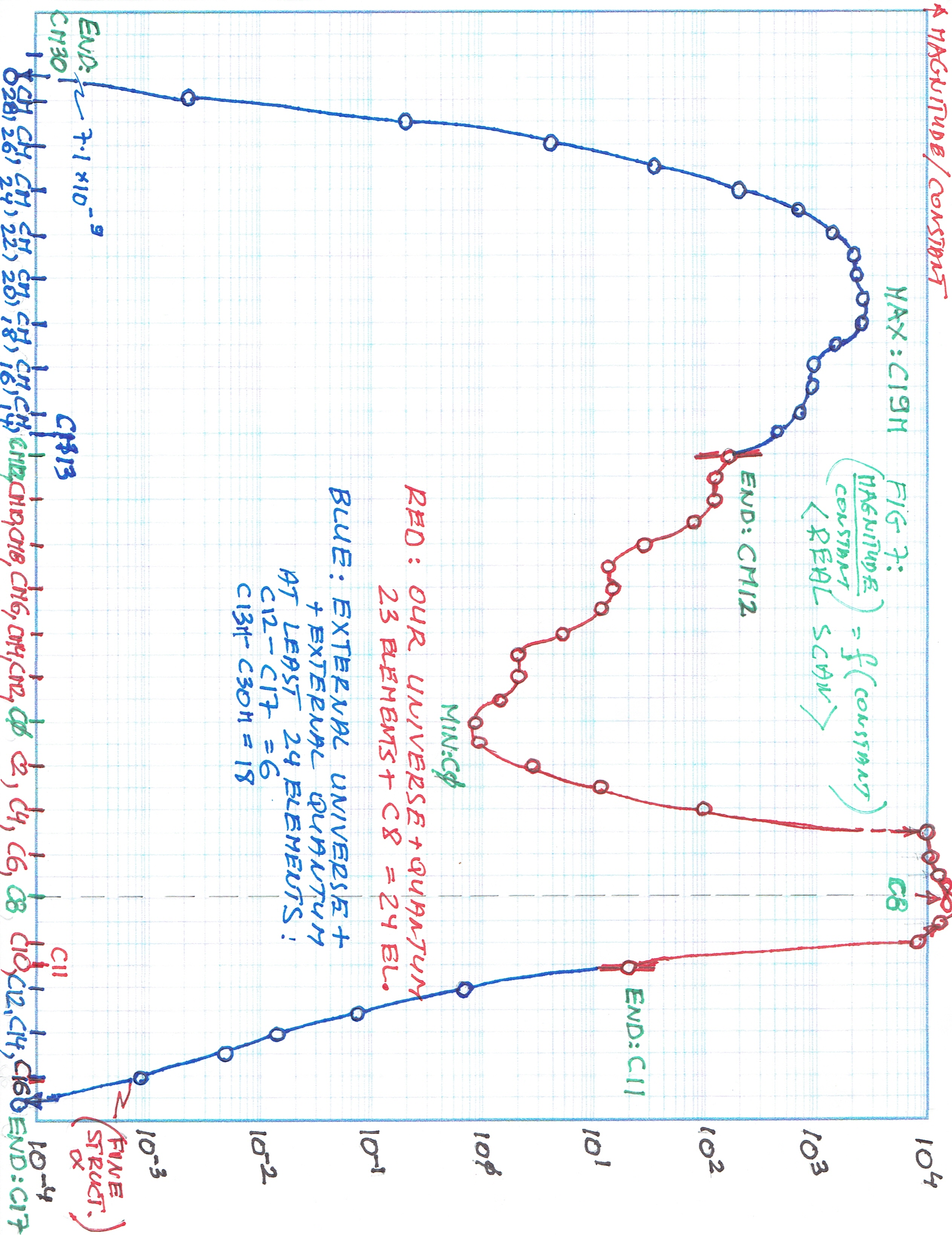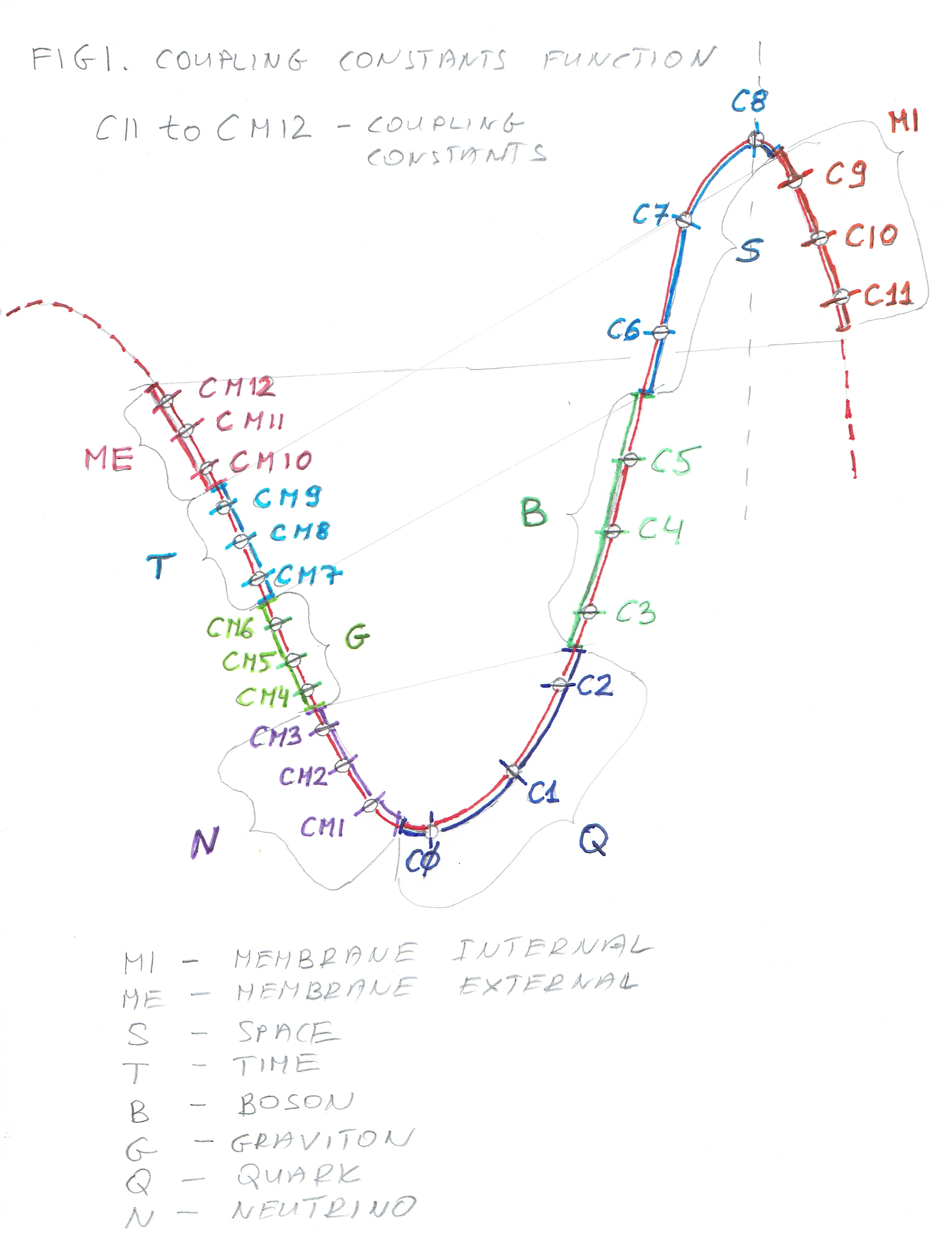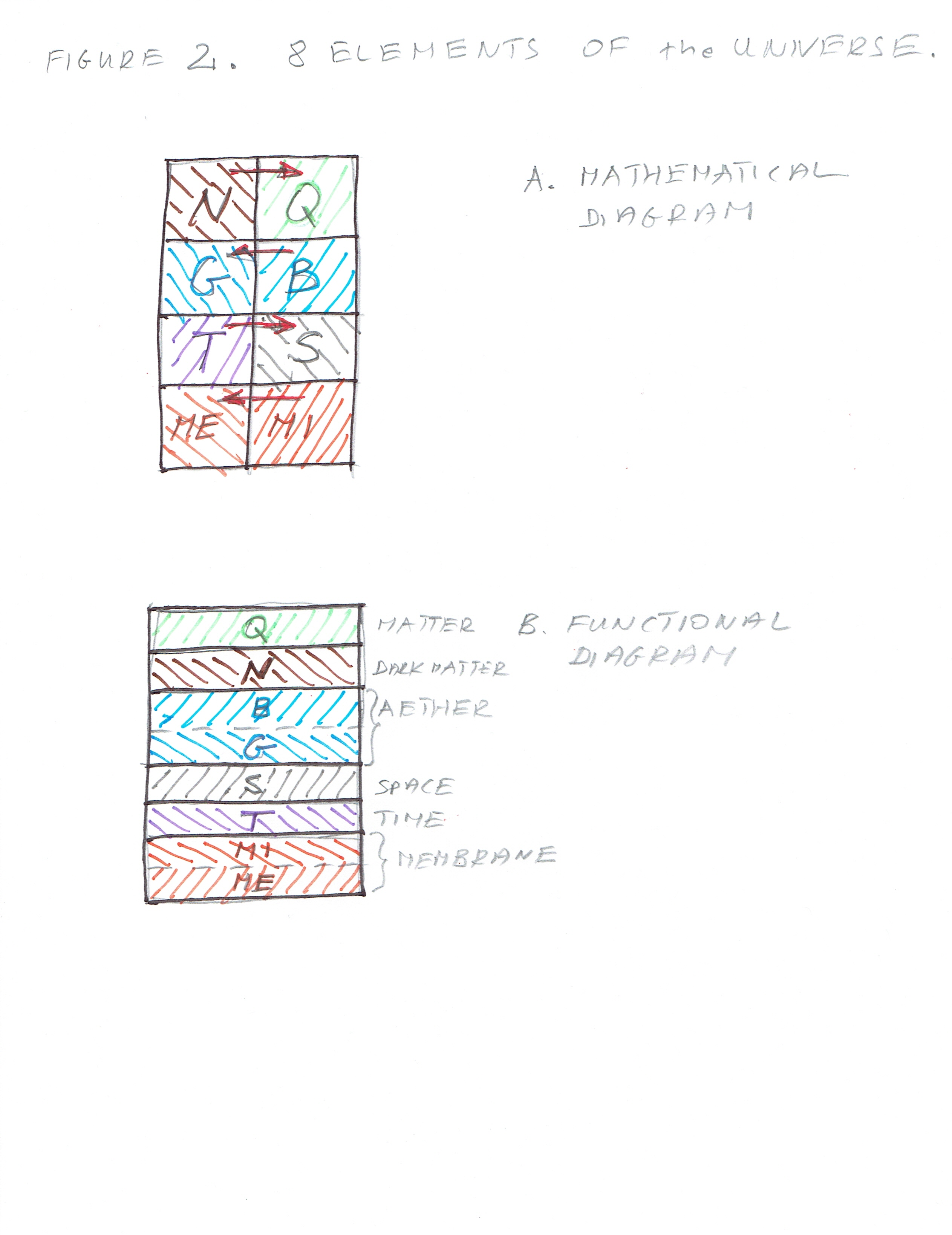St Blaise (316); St Ansgar (865)

Before I can derive all 8 Delta CP Violatining Phase Angles and the Sums of the Mixing Angles some introduction is necessary into the Structure of the Universe and in the next article the Transformation of the Mixing (Oscillation) Angles so the Sums of these angles can be calculated as well

From "Figure 7. - Graph of the Coupling Constants" can be seen that there are 24 Elements of the Universe divided into 8 sub-groups of three elements each. On the X-axis we have the Transcendental Constant and on Y-axis the value of the Coupling Constant. All delta CP Violating Phase Angles and Sigma - Sums of the Mixing (Oscillation) Angles, as well as the values of 4 Coupling Constants, are calculated from this graph.

The range of 24 elements is from Transcendental Constant CM12 (C Negative 12) to C11. Those values describe the Quantum Elements and at the same time, they describe precisely the structure of the Universe (more about it later).

Here is the graph (Fig. 7 - Graph of the Coupling Constants and Quantum Angles).On the second graph (Figure 1 - Coupling Constant Function) part of the same graph is shown. It describes Quantum Elements and at the same time the constituents of the Universe.

You can see MI (Membrane Internal) on the right side (C9, C10, C11) and ME (Membrane External) on the left side of the graph (CM10, CM11, CM12). These two are "merged" and form a unity.

Next are S (Space) and T (Time). Space is on the right side (C6, C7, C8) and Time is on the left (CM7, CM8, CM9). Those are not merged together.

Next pair of Elements consists of B (Boson) and G (Graviton). Boson and Graviton are again merged together and form unity - Aether. Boson is on the right side (C3, C4, C5) and Graviton on the left (CM4, CM5, CM6).

And lastly, there are Q (Quark) on the right side and N (Neutrino) on the left. Quark lies on the Transcendental Constants (C0, C1, C2) and Neutrino is on ((CM1, CM2, CM3).

Below there is the graph of the part of the function.On the third graph (Figure 2 - 8 Elements of the Universe) you can see two simple diagrams, "A. - Mathematical Diagram" and "B. - Functional Diagram". Mathematical Diagram is very important since it will be used to calculate all Delta CP Violating Phase Angles and Sigma - Sums of all Mixing (Oscillation) Angles for all Quantum Elements (or, if you prefer All Elements of the Universe).

There are certain relations between Delta CP Violating Phase Angles and Sigma Sums of Mixing Angles, but more about it later.

On the Functional Diagram, we can clearly see 8 Elements (Matter/Dark Matter/Aether/Space/Time/Membrane) grouped into 6 - Aether consists of (Boson and Graviton), and Membrane consists of Membrane Internal and membrane External as one unit.

St Hildegard von Bingen (1098-1179) -  (Hildegard of Bingen, also known as Saint Hildegard and the Sibyl of the Rhine, was a German Benedictine abbess, writer, composer, philosopher, Christian mystic, visionary, and polymath of the High Middle Ages. She is one of the best-known composers of sacred monophony, as well as the most-recorded in modern history.) describes a Vision which I will present in detail at the end of these series of articles where she (or rather God) talks about the structure of the Universe. From my calculation I have arrived at similar results i.e., it was obvious that there have to be more than 4 elements - there are two simple tests for that (again - later). So, Hildegard gave me quickly an idea of what to look for without wasting my time and being given the luxury of the "right guess" coming straight from the Creator.

Here is the last graph - Figure 2 - 8 elements of the Universe.In the next 2 articles, I will be writing about the Transformation necessary to calculate the Sigmas i.e. Sums of the Mixing (Oscillation) Angles.

After that, the real stuff will begin - getting all angles and more.